# KSEEB Solutions for Class 10 Maths Chapter 1 Arithmetic Progressions Ex 1.2

Students can Download Class 10 Maths Chapter 1 Arithmetic Progressions Ex 1.2 Questions and Answers, Notes Pdf, KSEEB Solutions for Class 10 Maths helps you to revise the complete Karnataka State Board Syllabus and to clear all their doubts, score well in final exams.

## Karnataka State Syllabus Class 10 Maths Chapter 1 Arithmetic Progressions Ex Ex 1.2

Question 1.
Fill in the blanks in the following table, given that a is first term, d the common difference and an the nth term of the A.P:

 a d n an (i) 7 3 8 … (ii) -18 … 10 0 (iii) … -3 18 -5 (iv) -18.9 2.5 … 3.6 (v) 3.5 0 105 …

(i) a = 7, d = 3, n = 8, an =?
an = a + (n – 1) d
a8= 7 + (8 – 1) 3
= 7 + 7 × 3
= 7 + 21
∴ a8 = 28(ii) a = -18, d =?, n = 10, an = 0
an = a + (n – 1) d
0 = -18 + (10 – 1) d
0 = -18 + 9d
18 = 9d
9d = 18
$$\mathrm{d}=\frac{18}{9}=2$$

(iii) a =?, d = -3, n = 18, an = -5
an = a + (n – 1) d
-5 = a + (18 – 1) (-3)
= a + 17(-3)
-6 = 1 – 51
∴ a = -5 + 51 = 46

(iv) a = -18.9, d = 2.5, n =? an = 3.6
an = a + (n – 1) d
3.6= -18.9 + (n – 1) (2.5)
3.6= -18.9 + 2.5n – 2.5
3.6 = 2.5n – 21.4
2.5n = 3.6 + 21.4
2.5n = 25
$$n=\frac{25}{2.5}=\frac{250}{25}$$
∴ n = 10.

(v) a = 3.5, d = 0, n = 105, an =?
an = a + (n – 1) d
= 3.5 + (105 – 1) (0)
= 3.5+ 104 × 0
= 3.5 +0
∴ an = 3.5

Least common multiple (LCM) of 12 and 18 is 36.

Question 2.
Choose the correct choice in the following and justify:
(i) 30th term of the A.P: 10, 7, 4,…. is
A) 97
B) 77
C) – 77
D) – 87
a = 10 an = a + (n – 1)d
d = a2 – a1 = 7 – 10, a30 10 + (30 – 1) – 3
d = – 3 a30 = 10 + 29 x – 3
n = 30, a30 = 10 – 87
a30 = ? a30 = – 77
Hence correct choice is C) – 77

(ii) 11th term of the AP: – 3, – $$\frac{1}{2}, 2$$ …. is
A) 28
B) 22
C) – 38
D) $$-48 \frac{1}{2}$$Hence the correct choice is B) 22LCM of 12 and 16 Calculator … LCM Calculator computes the LCM of two numbers i.e.

Question 3.
In the following APs, find the missing terms in the boxes: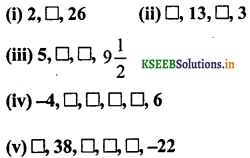(i)Common difference of an A.P is d.
a = 2 and a3 = 26
a3 a + 2d
26 = 2+2d
26 – 2 = 2d
2d = 24
d = $$\frac{24}{2}$$ = 12
d = 12
∴ Secondterm a2 = a + d2 + 12 = 14
Hence missing term in box = 14

ii)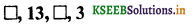Here, a =?, a + d = 13, a + 2d = ?
a + 3d = 3
a + d + 2d = 3
13 + 2d = 3
2d = 3 – 13
2d = -10
∴ d = -5
a + d = 13
a + (-5) = 13
a – 5 = 13
∴ a – 13 + 5 = 18
∴ a = 18
a + 2d =?
= 18 + 2(-5)
= 18 – 10
a + 2d = 8
∴ Ans: 18, 8

(iii)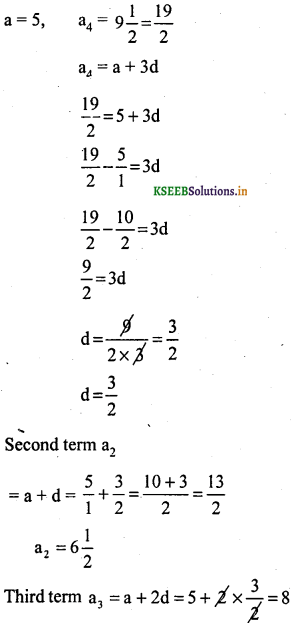(iv)a = -4, a +d=? a + 2d =?
a+3d = ? a + 4d =? a + 5d = 6
a + 5d = 6
-4 + 5d = 6
5d = 6 + 4
5d = 10
$$\mathrm{d}=\frac{10}{5}$$
∴ d = 2.
a + d = -4 + 2 = -2
a + 2d = -4 + 2 (2) = -4 + 4 = 0
a + 3d = -4 + 3 (2) = -4 + 6 = 2
a + 4d = -4 + 4 (2) = -4 + 8 = 4
∴ Ans: -2, 0, 2, 4

v)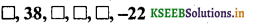a2 = 38 & a6 = – 22
a2 = a + d
38 = a + d
a = 38 – d ➝ (1)
a6 = a + 5d
– 22a + 5d ➝ (2)
Putting eqn (1) in eqn (2)
– 22 = 38 – d + 5d
– 22 – 38 = 4d
– 60 = 4d
d = $$\frac{-60}{4}$$ = – i5
d = – 15
Put d – 15 in eqn (1),
a = 38 – (- 15) = 38 + 15 = 53
First term = a = 53
Third term = a3 = a +2d = 53 + 2(- 15)
= 53 – 30 = 23
Fourth term = a4= a + 3d = 53 + 3(- 15)
= 53 – 45 = 8
First term = a5 = a + 4d = 53 + 4(- 15)
= 53 – 60 = – 7
Hence missing terms arc 53,23,8 & -7.

Question 4.
Which term of the AP: 3, 8, 13, 18, ……… is 78?
a = 3, d = 8 – 3 = 5, an = 78, n =?
an = a + (n – 1) d
78 = 3+(n—1)(5)
78 = 3 + 5n – 5
78 = 5n – 2
5n = 78 + 2
$$n=\frac{80}{5}$$
∴ n = 16Question 5.
Find the number of ternis in each of the following APs:
(i) 7, 13, 19, …., 205
(ii) 18, 15$$\frac{1}{2}$$, 13, ……. – 47
(i) 7, 13, 19, ………. 201
a = 3, d = 13 – 7 = 6, an = 201. n =?
a + (n – 1) d = an
3 + (n – 1) 6 = 201
3 + 6n – 6 = 201
6n – 3 = 201
6n = 203
$$n=\frac{204}{6}$$
∴ n = 34

(ii) Given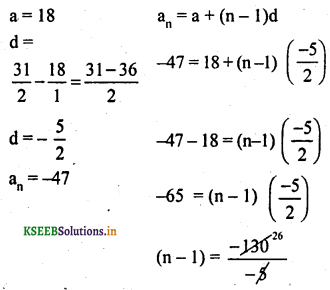n – 1 = 26
n = 26 + 1
n = 27
Hence, the number of terms of a given AP is 27

Question 6.
Check whether – 150 is the term of the AP: 11, 8, 5, 2, ….
11, 8, 5, 2, ……….. -150
a = 11, d = 8 11 = -3. an = -150.
a + (n – 1) d = an
11 + (n – 1) (-3) = -150
11 – 3n + 3 = -150
-3n + 14 = -150
-3n = -150 – 14
-3n = -164
3n = 164
$$n=\frac{164}{3}$$
Here value of ‘n’ is not perfect. Hence -150 is not a term of the A.P.

Question 7.
Find the 31st term of an AP whose 11th term is 38 and the 16th term is 73.
a11 = 38 & a16 = 73
a11 a + 10d
a = 38 – 10d ➝ (1)
a16 = a + 15d
73 = a + 15d ➝ (2)
Putting eqn (1) in eqn (2)
73 = 38 – 10d + 15d
73 – 38 = 5d.
35 = 5d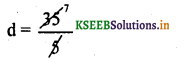d = 7.
Put d = 7 in eqn (1)
a = 38 – 10 x 7 = 38 – 70 = – 32
a = – 32
31st term a31 = a + 30d
a31 =- 32 + 30 x 7
= – 32 + 210
a31 = 178
Hence 31st term is 178.

Question 8.
An AP consists of 50 terms of which 3rd term is 12 and the last term is 106. Find the 29th term.
n = 50, a3 = 12, an = l06 & a29 = ?
a3 = a + 2d
12 = a + 2d
a = 12 – 2d ➝ (1)
a50= a + 49d
106 = a + 49d ➝ (2)
Putting eqn (1) in eqn (2)
106 = 12 – 2d + 49d
106 – 12 = 47d
94 = 47d
d = $$\frac{94}{47}$$ = 2
d = 2
Put d = 2 in eqn (1)
a = 12 – 2(2) 12 – 4 = 8
a = 8
a29 = a + 28d = 8 + 28(2) = 8 + 56 = 64
a29 = 64

Question 9.
If the 3rd and the 9th terms of an AP are 4 and 4 respectively, which term of this AP is zero?
a3 = 4 & a9= – 8, an = 0 & n = ?a3 + 2d
4 = a + 2(-2)
4 = a – 4
a = 4 + 4 = 8
a = 8 & d = – 2
an = a + (n – 1)d
0 = 8 +(n – 1)-2
0 = 8 -2n+2
2n = 10
n = $$\frac{110}{2}$$ = 5
Hence fifth term of an AP is zero

Question 10.
The 17th term of an AP exceeds its 10th term by 7. Find the common difference.
a17 = a10 + 7, d = ?
a + 16d = a + 9d + 7
a +1 6d – a – 9d = 7
7d = 7
$$\mathrm{d}=\frac{7}{7}$$ = 1Question 11.
Which term of the AP: 3, 15, 27, 39,… will be 132 more than Its 54th term?
a = 3, d = 15 – 3 = 12
an = a54 + 132
a + (n – 1)d = a + 53d + 132
3 + (n-1) 12 = 3 + 53(12) + 132
3 + 12n – 12 = 3 + 636 + 132
12n – 9 = 771
12n = 771 + 9
12n = 780n = 65
Hence 65th term will be 132 more than the 54th term.

Question 12.
Two APs have the same common difference. The difference between their 100th terms is 100, what is the difference between their 1000th terms?
Having common difference ‘d’, the APs
1st set a, a+d, a+2d
2nd set b, b + d, b + 2d
100th term of 1st set – 100th term of 2nd set = 100
∴ a + 99d – (b + 99d) = 100
a + 99d – b – 99d = 100
a – b= 100
Similarly.
1000th term of 1st set = 1 + 999d
1000 th term of 2nd set = b + 999d
Their difference
= a + 999d – (b + 999d)
= a + 999d – b – 999d
= a – b.

Question 13.
How many three-digit numbers are divisible by 7?
Let the three-digit numbers divisible by 7 are 105, 112, 119, …., 994
an = a + (n – 1) 105 d = 7, n = ?& an = 994
an = a + (n – 1)d
994 = 105 + (n – 1)7
994 – 105 = 7n – 7n = 128
Hence there are 128 three-digit numbers divisible by 7.

Question 14.
How many multiples of 4 11e between 10 and 250?
Let the multiples of 4 between 10 and 250 are 12, 16,20 …….. 248
a = 12, d = 4, a = 248 and n = ?
a = a + (n – 1)d
248 = 12 + (n – 1)4
248 – 12 = 4n – 4
236 + 4 = 4n
4n = 240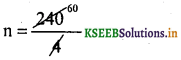n = 60
Hence 60 multiples lie between 10 and 250 multiples of 4

Question 15.
For what value of n, are the nth terms of two APs: 63, 65, 67,… and 3, 10, 17,… equal?
63, 65, 67,……….
a = 63. d = 65 – 63 =2. an =?
nth term of this is
an = a + (n – 1)d
= 63 + (n – 1) 2
= 63 + 2n – 2
an= 2n + 61 …………….. (i)
3, 10, 17, ………….
a = 3, d = 10 – 3 = 7, an =?
an = a + (n – 1)d
= 3 + (n – 1) 7
= 3 + 7n – 7
an = 7n — 4 ………….(ii)
Here. nth terms of second AP are equal.
∴ equation (i) = equation (ii)
2n + 61 = 7n – 4
2n – 7n = -4 – 61
5n = 65
5n =65
$$n=\frac{65}{5}$$
∴ n = 13
∴13th terms of the two given APs are equal.

Question 16.
Determine the AP whose third term Is 16 and the 7th term exceeds the 5th term by 12.
a = 16, a7 = a5 + 12, A.P =?
a7 = a5 + 12
a + 6d = a + 4d + 12
a + 6d – a – 4d = 12
2d = 12 ∴ d = 6.
a + 2d = 16
a + 2(6) = 16
a + 12 = 16
∴ a = 16 – 12 ∴ a = 4
a = 4, d = 6.
∴ A.P.:
a, a + d, a + 2d, …………………
4, 4 + 6, 4 + 12, …………….
4, 10, 16, ………….Question 17.
Find the 20th term from the last term of the AP: 3, 8, 13, …., 253.
3, 8, 13, ………….., 253
a = 3. d = 8 – 3 = 5, an = 253
20th term from the last term of the AP starting from 253 =?
253, 258, 263, ………… a20 =?
a = 253, d = 258 – 253 = 5, n = 20
an = a + (n – 1) d
a20= 253 + (20 – 1) 5
= 253 + 19 × 5
= 253 + 95
∴ a20 = 348
∴ 20th term from the last term of the AP is 348.

Question 18.
The sum of the 4th and 8th terms of an AP is 24 and the sum of the 6th and 10th terms is 44. Find the first three terms of the AP.
a4 + a8 = 24
a + 3d + a + 7d = 24
2a + 10d = 24 divideby 2
a + 5d = 12
a = 12 – 5d ➝ (1)
a6 + a10 = 44
a + 5d + a + 9d = 44
2a + 14d = 44 divide by 2
a + 7d = 22
Put eqn (2) in eqn (1)
12 – 5d + 7d = 22
2d = 22 – 12
2d = 10
d = $$\frac{10}{2}$$ = 5
Put d = 5 in eqn (1)
a = 12 – 5 (5) 12 – 25
a = – 13
First three terms an A.P a, a + d, a + 2d
– 13, – 13 + 5, – 13 + 2(5)
– 13, – 8, – 3
∴Three terms are – 13, 4, -3

Question 19
Subba Rao started work in 1995 at an annual salary of ₹ 5000 and received an increment of ₹ 200 each year. In which year did his income reach ₹ 7000?
The annual salary in 1995 = ₹ 5000
annual salary in 1996 = ₹ 5200
∴ AP, 5000, 5200, 5400,…, 7000.
Given:
a = 5000 an = a + (n – 1)d
d = 5200 – 5000, 7000 = 5000 + (n – 1)200 = 200
n = ?, 7000 – 5000 = (n – 1)200
an = 7000
(n – 1) = $$\frac{2000}{200}$$ = 10
n – 1 = 10
n = 10 + 1 = 1
n = 11
Subba Rao annual salaiy will be ₹ 7000 after 11 years.
∴ In the year 2005.

Question 20.
Ramkali saved ₹ 5 in the first week of a year and then increased her weekly savings by ₹ 1.75. If in the nth week, her weekly savings become ₹ 20.75, find n.
Savings in the First week = Rs. 5.
Savings in the Second week 5 + 1.75 = Rs. 6.75
Savings in ‘nth week is Rs. 20.75
∴ A.P. 5, 6, 75, …………., 20.75
a = 5, d = 6.75 – 5 = 1.75, an = 20.75.
n =?
a + (n – 1) d = an
5 + (n – 1) (1.75) = 20.75
5 + 1.75n – 1.75 = 20.75
1 .75n + 3.25 = 20.75
1.75n = 20.75 – 3.25
1.75n = 17.5
$$n=\frac{17.5}{1.75}$$
$$n=\frac{1750}{175}$$
∴ n = 10
∴ In the 10th week, her savings becomes Rs. 20.75.